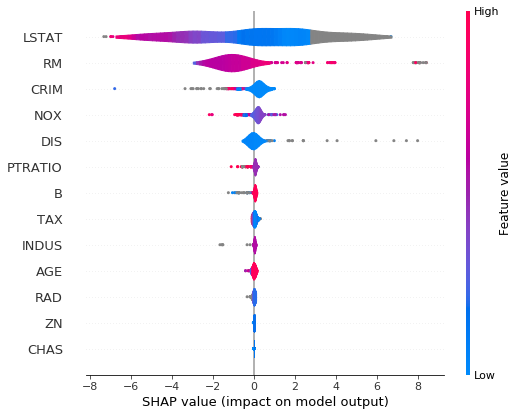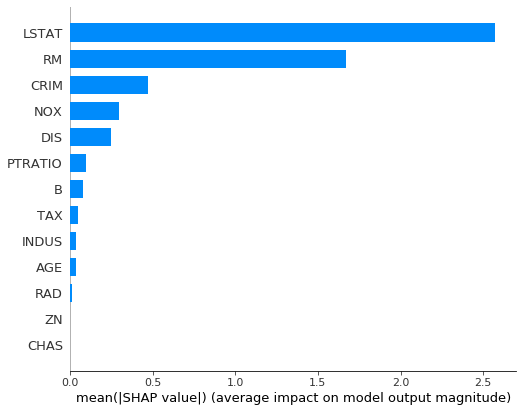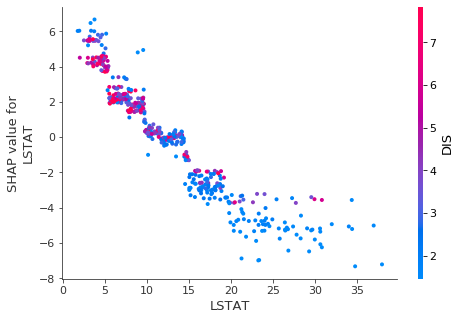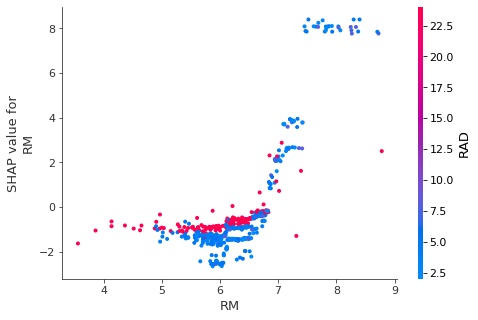# Interpretability of prediction for Boston Housing using SHAP

## Goal¶

This post aims to introduce how to interpret the prediction for Boston Housing using shap.

What is SHAP?

SHAP is a module for making a prediction by some machine learning models interpretable, where we can see which feature variables have an impact on the predicted value. In other words, it can calculate SHAP values, i.e., how much the predicted variable would be increased or decreased by a certain feature variable.

Reference

## Libraries¶

In :
import xgboost
import shap
shap.initjs()In :
X, y = shap.datasets.boston()
X[:5]

Out:
CRIM ZN INDUS CHAS NOX RM AGE DIS RAD TAX PTRATIO B LSTAT
0 0.00632 18.0 2.31 0.0 0.538 6.575 65.2 4.0900 1.0 296.0 15.3 396.90 4.98
1 0.02731 0.0 7.07 0.0 0.469 6.421 78.9 4.9671 2.0 242.0 17.8 396.90 9.14
2 0.02729 0.0 7.07 0.0 0.469 7.185 61.1 4.9671 2.0 242.0 17.8 392.83 4.03
3 0.03237 0.0 2.18 0.0 0.458 6.998 45.8 6.0622 3.0 222.0 18.7 394.63 2.94
4 0.06905 0.0 2.18 0.0 0.458 7.147 54.2 6.0622 3.0 222.0 18.7 396.90 5.33
In :
y[:5]

Out:
array([24. , 21.6, 34.7, 33.4, 36.2])

## Train a predictor by xgboost¶

In :
d_param = {
"learning_rate": 0.01
}

model = xgboost.train(params=d_param,
dtrain=xgboost.DMatrix(X, label=y),
num_boost_round=100)


## Create an explainer¶

In :
explainer = shap.TreeExplainer(model)
shap_values = explainer.shap_values(X)


## Outcome of SHAP¶

### Single prediction explainer¶

The visualization below shows the explanations for one prediction based on i-th data.

• red: positive impacts on the prediction
• blue: negative impacts on the prediction
In :
i = 0
shap.force_plot(explainer.expected_value, shap_values[i,:], X.iloc[i,:])

Out:
Visualization omitted, Javascript library not loaded!
Have you run initjs() in this notebook? If this notebook was from another user you must also trust this notebook (File -> Trust notebook). If you are viewing this notebook on github the Javascript has been stripped for security. If you are using JupyterLab this error is because a JupyterLab extension has not yet been written.

### All prediction explainers¶

All explainers like the above are plotted in one graph as below.

In :
shap.summary_plot(shap_values, X, plot_type="violin")### Variable importance¶

This variable importance shown as below simply aggregates the above by computing the sum of the absolute values of shap values for all data points.

In :
shap.summary_plot(shap_values, X, plot_type="bar")### Force Plot¶

The other way of visualizing shap values are the one to stack all shap values across samples or feature values themselves.

In :
shap.force_plot(explainer.expected_value, shap_values, X)

Out:
Visualization omitted, Javascript library not loaded!
Have you run initjs() in this notebook? If this notebook was from another user you must also trust this notebook (File -> Trust notebook). If you are viewing this notebook on github the Javascript has been stripped for security. If you are using JupyterLab this error is because a JupyterLab extension has not yet been written.

### Dependency Plot¶

This plot shows a certain value and its shap value as a scatter plot with the color specified by automatically selected variable, which separates most the certain value and its shap value.

In :
# specify by the index of the features
shap.dependence_plot(ind=12, shap_values=shap_values, features=X)In :
# specify by the feature name
shap.dependence_plot(ind="RM", shap_values=shap_values, features=X)